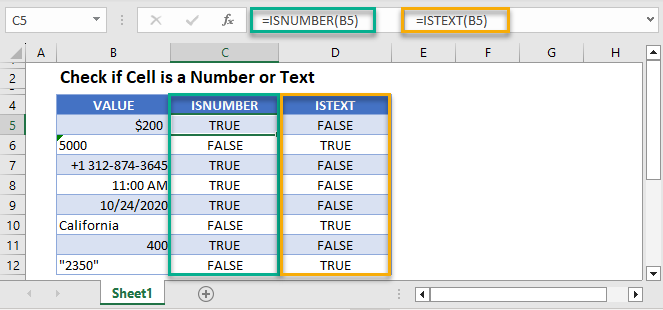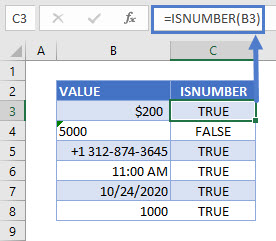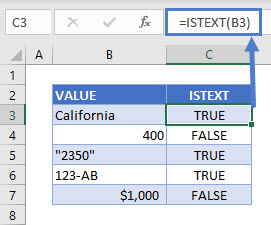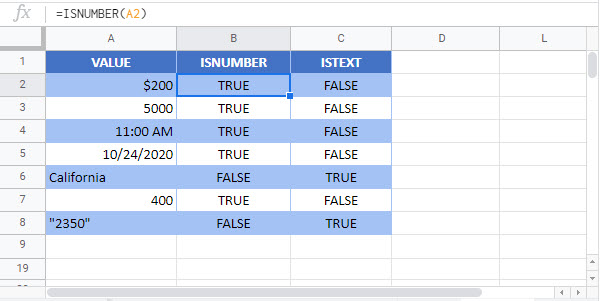# Check if Cell is a Number or Text – Excel & Google Sheets

This tutorial demonstrates how to check if a cell is a number or text in Excel and Google Sheets.## Check Cell for Number or Text

We can check if a cell is a number by using the ISNUMBER Function or check if a cell is text with the ISTEXT Function.

### ISNUMBER Function

The ISNUMBER Function checks if a value is a number. It returns TRUE if the value is a number and FALSE if it’s not.

``=ISNUMBER(B3)``Knowing if a cell value is a number helps avoid errors when working with formulas. Numbers are sometimes stored as text, i.e. B4 (5000) in the example above, and that can result in errors in formulas referencing that cell.

In this example, the date, phone number, and time values all returned TRUE. These are regarded as numeric values in Excel and Google Sheets.

### ISTEXT Function

The ISTEXT Function checks if a cell is text. It returns TRUE if the value is a text string and FALSE if it’s not.

``=ISTEXT(B3)``In this example, although the value in B5 (2350) is a number, it’s written within quotes and therefore stored as a text string. That’s why it returns TRUE.

## Check if Cell is a Number or Text in Google Sheets

These formulas (ISNUMBER and ISTEXT) work exactly the same in Google Sheets as in Excel.### Excel Practice Worksheet

Practice Excel functions and formulas with our 100% free practice worksheets!